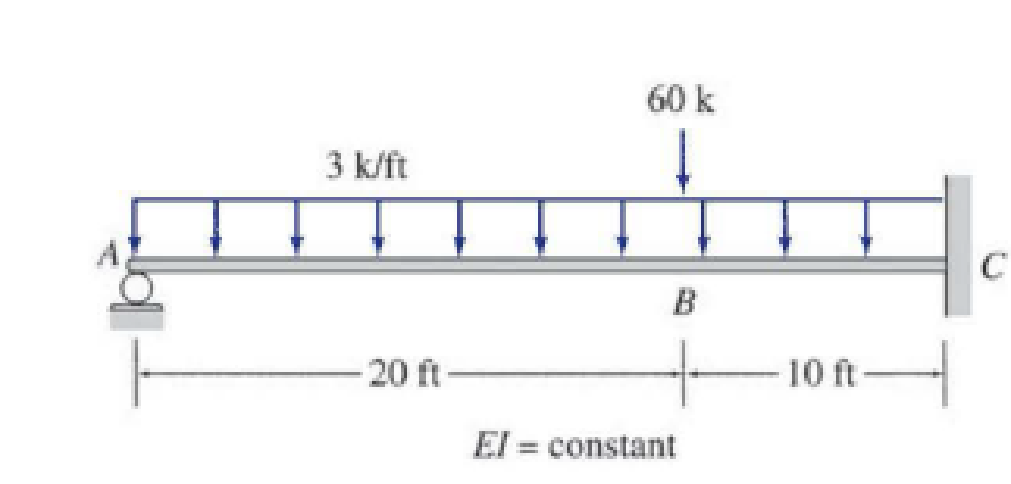# Determine the reactions and draw the shear and bending moment diagrams for the beams shown in Figs. P13.1–P13.4 using the method of consistent deformations. Select the reaction at the roller support to be the redundant.

#### Solutions

Chapter
Section
Chapter 13, Problem 4P
Textbook Problem
308 views

## Determine the reactions and draw the shear and bending moment diagrams for the beams shown in Figs. P13.1–P13.4 using the method of consistent deformations. Select the reaction at the roller support to be the redundant.To determine

Find the reactions and sketch the shear and bending moment diagrams for the given beam using method of consistent deformation.

### Explanation of Solution

Given information:

Apply the sign conventions for calculating reactions, forces and moments using the three equations of equilibrium as shown below.

• For summation of forces along x-direction is equal to zero (Fx=0), consider the forces acting towards right side as positive (+) and the forces acting towards left side as negative ().
• For summation of forces along y-direction is equal to zero (Fy=0), consider the upward force as positive (+) and the downward force as negative ().
• For summation of moment about a point is equal to zero (Matapoint=0), consider the clockwise moment as negative and the counter clockwise moment as positive.

Calculation:

Show the beam as shown in the Figure 1.

Refer Figure 1.

Consider the horizontal and vertical reaction at C are denoted by Cx and Cy.

Consider the moment at C is denoted by MC.

Consider the vertical reaction at A is denoted by Ay.

The reactions acting in the beam is 4.

The number of Equilibrium reaction is 3. Then,

The degree of indeterminacy of the beam is 1.

Take the vertical reaction at A as the redundant.

Modify the Figure 1 as shown in Figure 2.

Refer Figure 1 and Figure 2.

The deflection at A due to the external force denoted by ΔAO.

The deflection coefficient representing the upward deflection at A due to unit value of redundant Ay is fAA. Then,

The deflection at B due to unknown redundant Ay is fAAAy.

Show the deflection at the free end of the cantilever beam with a point load P acting at free end as follows:

Δ=PL33EI        (1)

Here, L is the length of the beam, E is modulus of Elasticity of the beam, I is the moment of inertia.

Calculate the value of fAA as follows:

Substitute 1k for P and 30ft for L in Equation (1).

fAA=1×3033EI=9000kft3/kEI        (2)

Show the deflection at the free end of the cantilever beam due to the uniformly distributed load w as follows:

Δ1=wL48EI

Substitute 3k/ft for w and 30ft for L.

Δ1=3×3048EI=303,750kft38EI

Show the deflection at the free end of the cantilever beam with a point load P acting at a distance from the fixed end as follows:

Δ=Pa26EI(3La)        (3)

Here, l is the length of the beam, E is modulus of Elasticity of the beam, I is the moment of inertia.

Find the deflection at the free end of the beam due to the load of 60k as follows:

Substitute 10ft for a, 30ft for L and 60k for P in Equation (3).

Δ2=60×1026EI(3×3010)Δ2=80,000kft3EI

Calculate the deflection at A due to the loading on the beam as follows:

ΔAO=Δ1+Δ2=303,7508EI+80,000EI=383,750kft3EI        (4)

Show the compatibility Equation as follows:

ΔAO+fAA×Ay=0

Modify the above Equation using Equation (2) and (4)

### Still sussing out bartleby?

Check out a sample textbook solution.

See a sample solution

#### The Solution to Your Study Problems

Bartleby provides explanations to thousands of textbook problems written by our experts, many with advanced degrees!

Get Started

Find more solutions based on key concepts
What are the major components of a building?

Engineering Fundamentals: An Introduction to Engineering (MindTap Course List)

Differentiate between volatile and nonvolatile memory. List an example of each.

Enhanced Discovering Computers 2017 (Shelly Cashman Series) (MindTap Course List)

What should you do if you have to leave a piece of hot metal unattended?

Welding: Principles and Applications (MindTap Course List)

If your motherboard supports ECC DDR3 memory, can you substitute non-ECC DDR3 memory?

A+ Guide to Hardware (Standalone Book) (MindTap Course List)